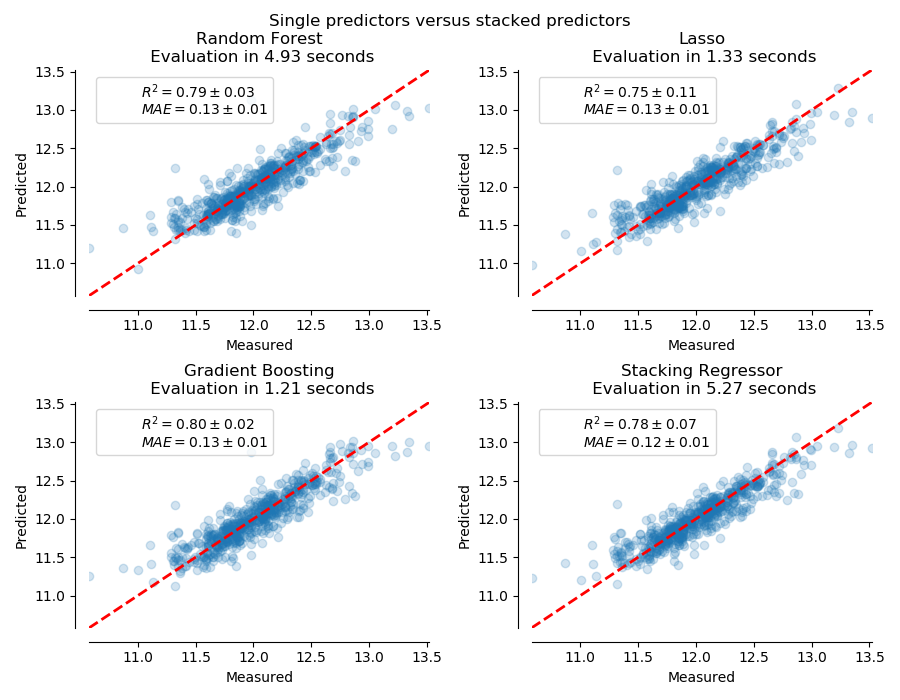# sklearn.ensemble.StackingRegressor¶

class sklearn.ensemble.StackingRegressor(estimators, final_estimator=None, *, cv=None, n_jobs=None, passthrough=False, verbose=0)

[源码]

0.22版本新功能。

estimators list of (str, estimator)

final_estimator estimator, default=None

cv int, cross-validation generator or an iterable, default=None

- None，使用默认的5折交叉验证。
- Integer, 用于指定(分层的)K-Fold中的折叠数。
- 用作交叉验证生成器的对象。
- 一个可迭代的产生的训练、测试分割。

n_jobs int, default=None

passthrough bool, default=False

verbose int, default=0

estimators_ list of estimators

named_estimators_ Bunch

final_estimator_ estimator

Wolpert, David H. “Stacked generalization.” Neural networks 5.2 (1992): 241-259.

>>> from sklearn.datasets import load_diabetes>>> from sklearn.linear_model import RidgeCV>>> from sklearn.svm import LinearSVR>>> from sklearn.ensemble import RandomForestRegressor>>> from sklearn.ensemble import StackingRegressor>>> X, y = load_diabetes(return_X_y=True)>>> estimators = [...     ('lr', RidgeCV()),...     ('svr', LinearSVR(random_state=42))... ]>>> reg = StackingRegressor(...     estimators=estimators,...     final_estimator=RandomForestRegressor(n_estimators=10,...                                           random_state=42)... )>>> from sklearn.model_selection import train_test_split>>> X_train, X_test, y_train, y_test = train_test_split(...     X, y, random_state=42... )>>> reg.fit(X_train, y_train).score(X_test, y_test)0.3...

fit(X[, y, sample_weight]) 拟合估计器。
fit_transform(X[, y]) 拟合估计器和变换数据集。
get_params([deep]) 从集成中得到估计器的参数。
predict(X, **predict_params) 预测X的目标值。
score(X, y[, sample_weight]) 返回给定测试数据和标签的平均精度。
set_params(**params) 从集成中设置估计器的参数。
transform(X) 返回每个估计器X的类标签或概率。
__init__(estimators, final_estimator=None, *, cv=None, n_jobs=None, passthrough=False, verbose=0)

[源码]

fit(X, y, sample_weight = None)

[源码]

X {array-like, sparse matrix} of shape (n_samples, n_features)

y array-like of shape (n_samples,)

sample_weight array-like of shape (n_samples,), default=None

self object
fit_transform(X, y=None, **fit_params)

[源码]

X {array-like, sparse matrix} of shape (n_samples, n_features)

y ndarray of shape (n_samples,), default=None

**fit_params dict

X_new ndarray array of shape (n_samples, n_features_new)

get_params(deep=True)

[源码]

deep deep : bool, default = True

property n_features_in_

fit 过程中可见的特征数量。

predict(X, **predict_params)

[源码]

X {array-like, sparse matrix} of shape (n_samples, n_features)

**predict_params dict of str -> obj
final_estimator调用的predict的参数。请注意，这可能用于从一些使用return_stdreturn_cov的估计器返回不确定性。请注意，它只会在最终的估计器中考虑不确定性。

y_pred ndarray of shape (n_samples,) or (n_samples, n_output)

score(X, y, sample_weight=None)

[源码]

X array-like of shape (n_samples, n_features)

y array-like of shape (n_samples,) or (n_samples, n_outputs)
X的正确值。
sample_weight array-like of shape (n_samples,), default=None

score float
self.predict(X) 关于y的平均准确率。

set_params(**params)

[源码]

**params keyword arguments

transform(X)

[源码]

X {array-like, sparse matrix} of shape (n_samples, n_features)

y_preds ndarray of shape (n_samples, n_estimators) or (n_samples, n_classes * n_estimators)

## sklearn.ensemble.StackingRegressor使用示例¶